# RD Sharma Solutions for Class 9 Maths Chapter 1 Number System Exercise 1.5

RD Sharma Class 9 Mathematics Chapter 1 Exercise 1.5 Number System is provided here. This exercise helps students to understand and practice irrational numbers and representation of real numbers and irrational numbers on the number line. RD Sharma Solutions Class 9 chapter 1 are designed by experts in accordance with CBSE syllabus for 9th standard.

## Download PDF of RD Sharma Solutions for Class 9 Maths Chapter 1 Number System Exercise 1.5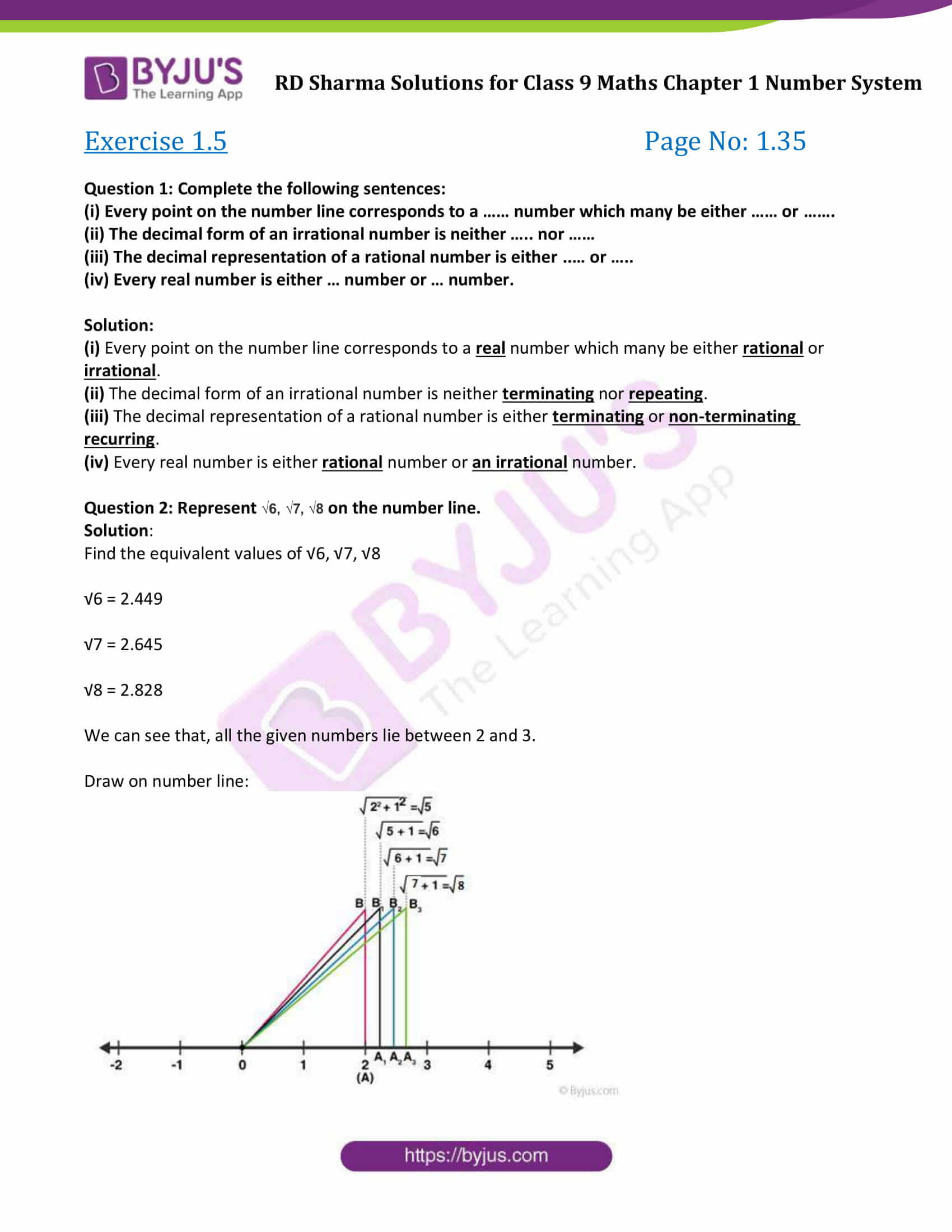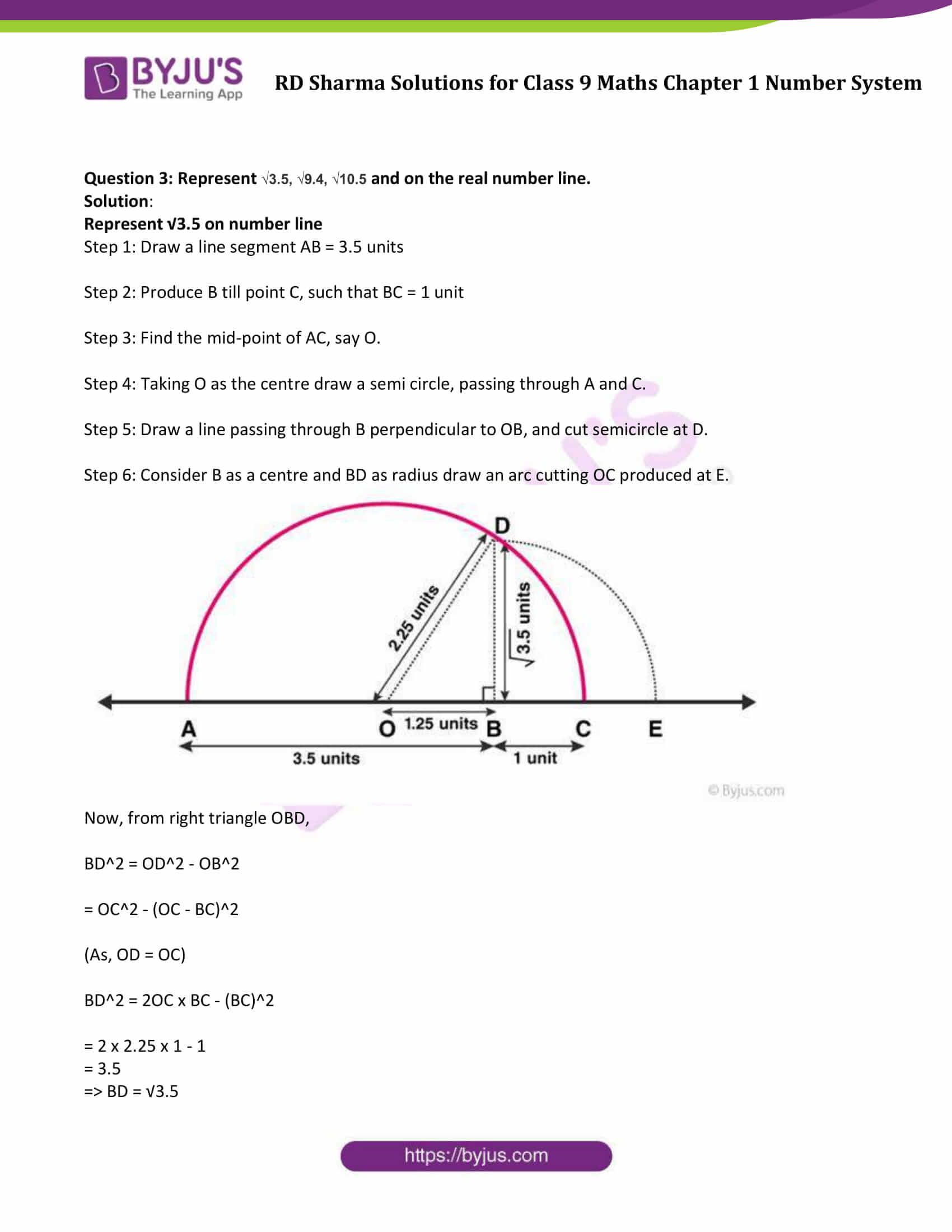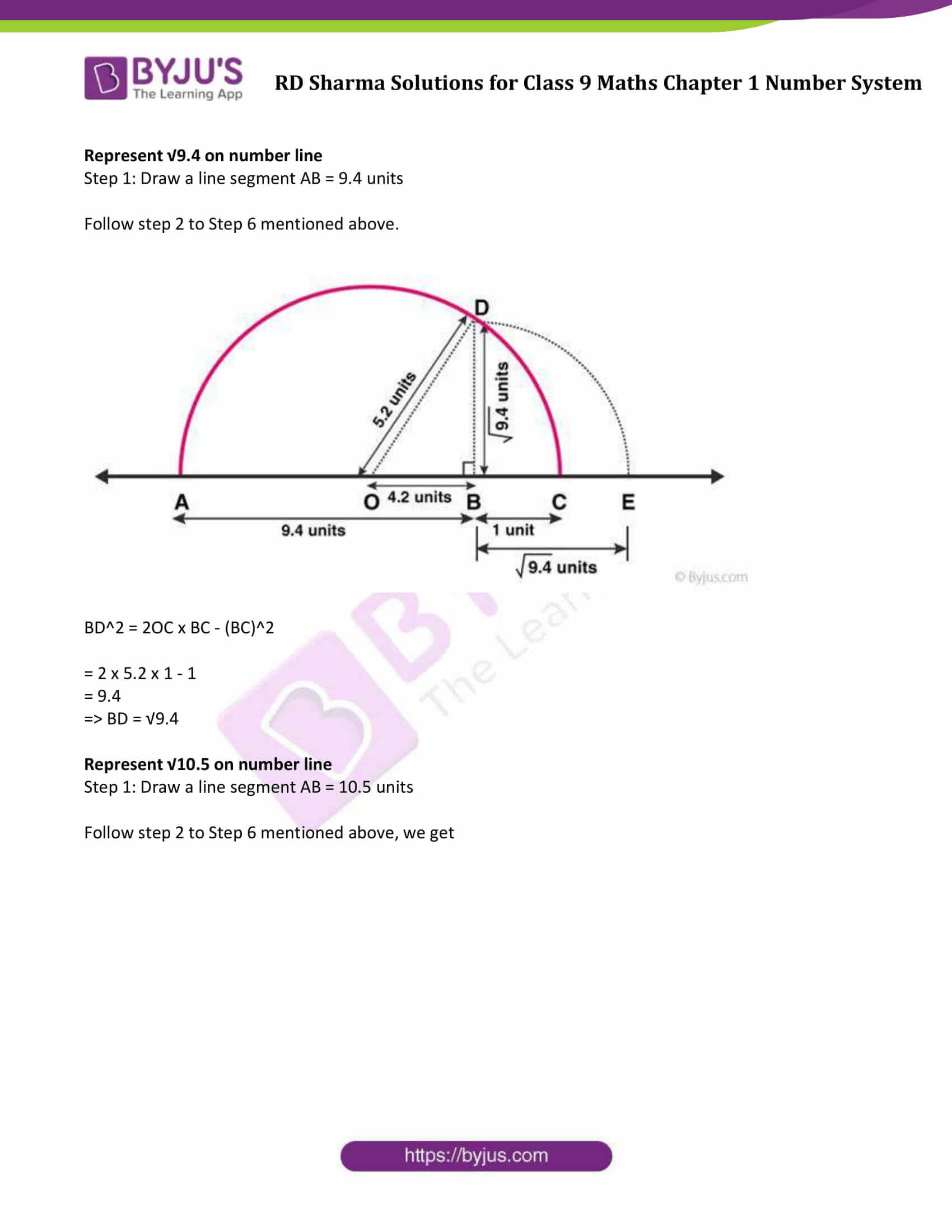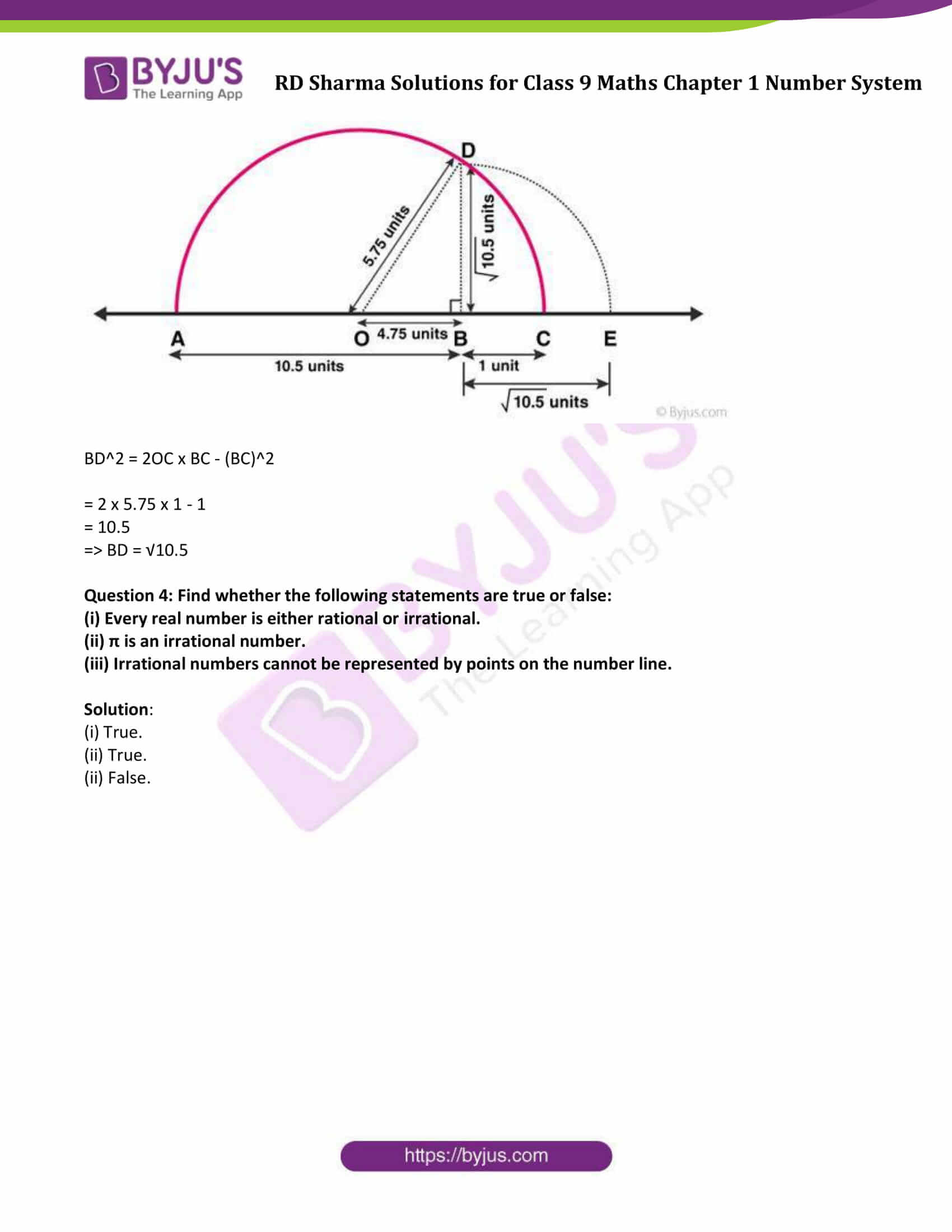### Access Answers to Maths RD Sharma Solutions for Class 9 Chapter 1 Number System Exercise 1.5

Question 1: Complete the following sentences:

(i) Every point on the number line corresponds to a …… number which many be either …… or …….

(ii) The decimal form of an irrational number is neither ….. nor ……

(iii) The decimal representation of a rational number is either ..… or …..

(iv) Every real number is either … number or … number.

Solution:

(i) Every point on the number line corresponds to a real number which many be either rational or irrational.

(ii) The decimal form of an irrational number is neither terminating nor repeating.

(iii) The decimal representation of a rational number is either terminating or non-terminating recurring.

(iv) Every real number is either rational number or an irrational number.

Question 2: Represent √6, √7, √8 on the number line.

Solution:

Find the equivalent values of √6, √7, √8

√6 = 2.449

√7 = 2.645

√8 = 2.828

We can see that, all the given numbers lie between 2 and 3.

Draw on number line: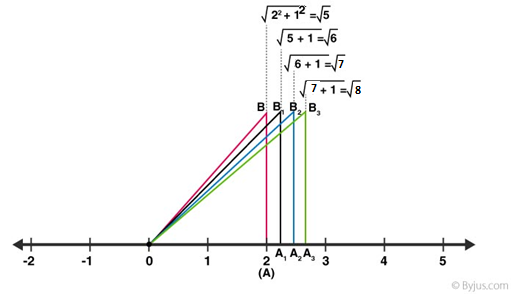Question 3: Represent √3.5, √9.4, √10.5 and on the real number line.

Solution:

Represent √3.5 on number line

Step 1: Draw a line segment AB = 3.5 units

Step 2: Produce B till point C, such that BC = 1 unit

Step 3: Find the mid-point of AC, say O.

Step 4: Taking O as the centre draw a semi circle, passing through A and C.

Step 5: Draw a line passing through B perpendicular to OB, and cut semicircle at D.

Step 6: Consider B as a centre and BD as radius draw an arc cutting OC produced at E.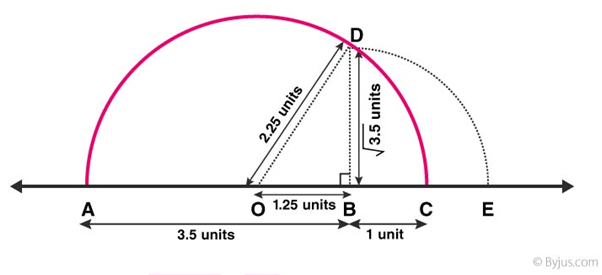Now, from right triangle OBD,

BD2 = OD2 – OB2

= OC2 – (OC – BC)2

(As, OD = OC)

BD2 = 2OC x BC – (BC)2

= 2 x 2.25 x 1 – 1

= 3.5

=> BD = √3.5

Represent √9.4 on number line

Step 1: Draw a line segment AB = 9.4 units

Follow step 2 to Step 6 mentioned above.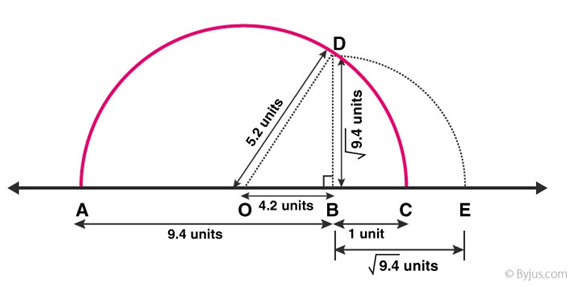BD2 = 2OC x BC – (BC)2

= 2 x 5.2 x 1 – 1

= 9.4

=> BD = √9.4

Represent √10.5 on number line

Step 1: Draw a line segment AB = 10.5 units

Follow step 2 to Step 6 mentioned above, we get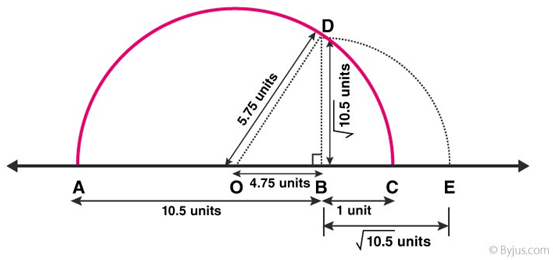BD2 = 2OC x BC – (BC)2

= 2 x 5.75 x 1 – 1

= 10.5

=> BD = √10.5

Question 4: Find whether the following statements are true or false:

(i) Every real number is either rational or irrational.

(ii) π is an irrational number.

(iii) Irrational numbers cannot be represented by points on the number line.

Solution:

(i) True.

(ii) True.

(ii) False.